Ch 6. Entropy Multimedia Engineering Thermodynamics Entropy Tds Relations EntropyChange IsentropicProcess IsentropicEfficiency EntropyBalance (1) EntropyBalance (2) ReversibleWork
 Chapter 1. Basics 2. Pure Substances 3. First Law 4. Energy Analysis 5. Second Law 6. Entropy 7. Exergy Analysis 8. Gas Power Cyc 9. Brayton Cycle 10. Rankine Cycle Appendix Basic Math Units Thermo Tables Search eBooks Dynamics Fluids Math Mechanics Statics Thermodynamics Author(s): Meirong Huang Kurt Gramoll ©Kurt GramollTHERMODYNAMICS - CASE STUDY SOLUTION The entropy generated when Austin's father takes his daily shower needs to be determined. Assumptions: The shower operates steadily at maximum flow condition. Heat loss from the pipes is negligible. Water is an incompressible substance with a density equals 1000 kg/m3 and a specific heat equals 4.18 kJ/(kg-K). The kinetic and potential energies are negligible.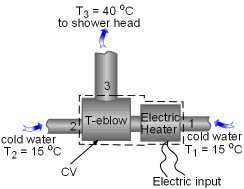Take the T-elbow and the Electric Heater together as a Control Volume Take the T-elbow and the electric heater together as a control volume shown on the left. Cold water at 15oC enters the electric heater (denoted as 1) and the T-elbow (denoted as 2), and leaves the T-elbow at 40oC. Since the flow is steady, the mass balance for this control volume is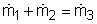The mass flow rate to the shower head is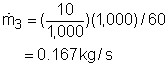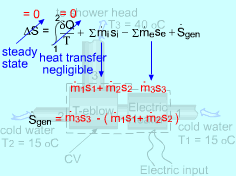Simplify the Entropy Balance of the Control Volume The entropy balance for a control volume at steady state is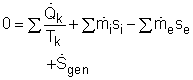It is assumed that there is no heat transfer between the pipes and the surroundings. That means no entropy flows in or out of the control volume associated with the heat transfer. The control volume has electric work input, but the work is entropy free. Hence, the entropy balance for this steady-flow control volume can be simplified as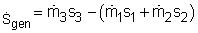Since water enters the T-elbow and the electric heater at the same temperature,       s1 = s2 Also, with the mass balance, the entropy generation can be determined by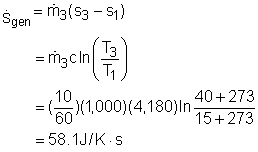Hence, a 15-minute shower will generate entropy       Sgen = (15)(60)(58.1) = 52,290 J/K = 52.3 kJ/K